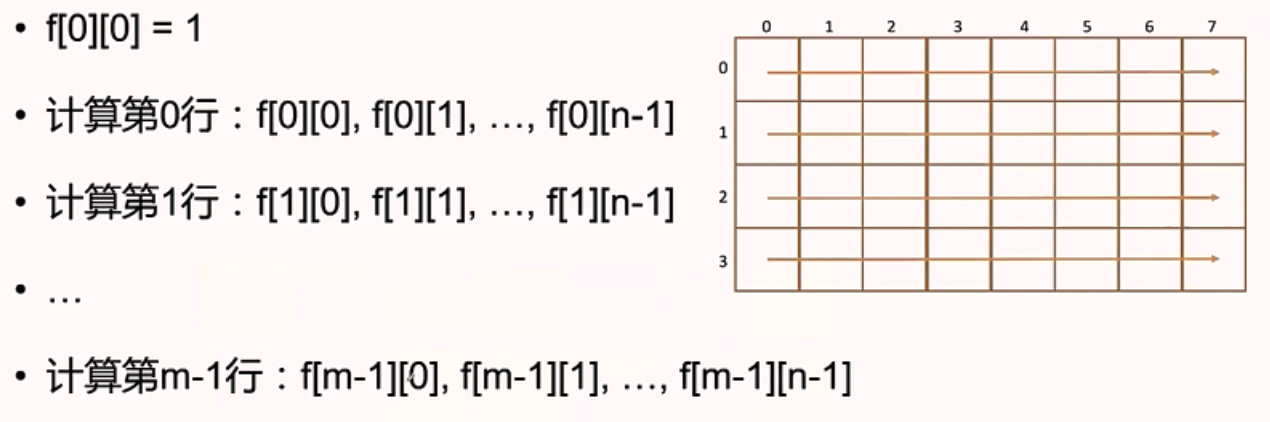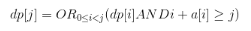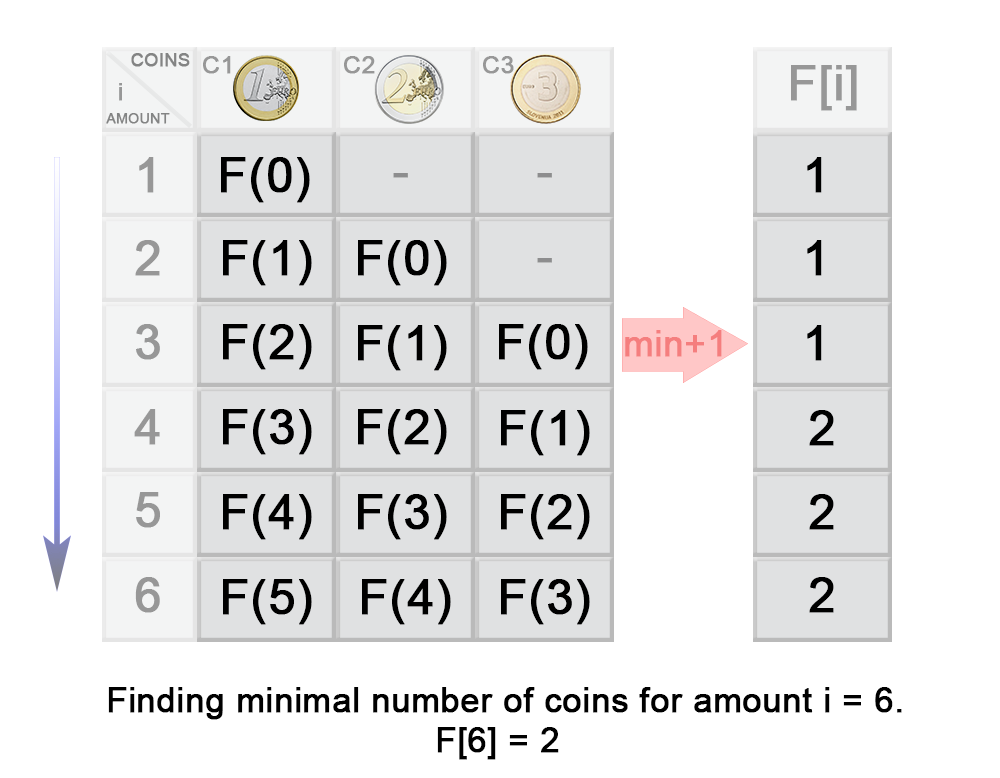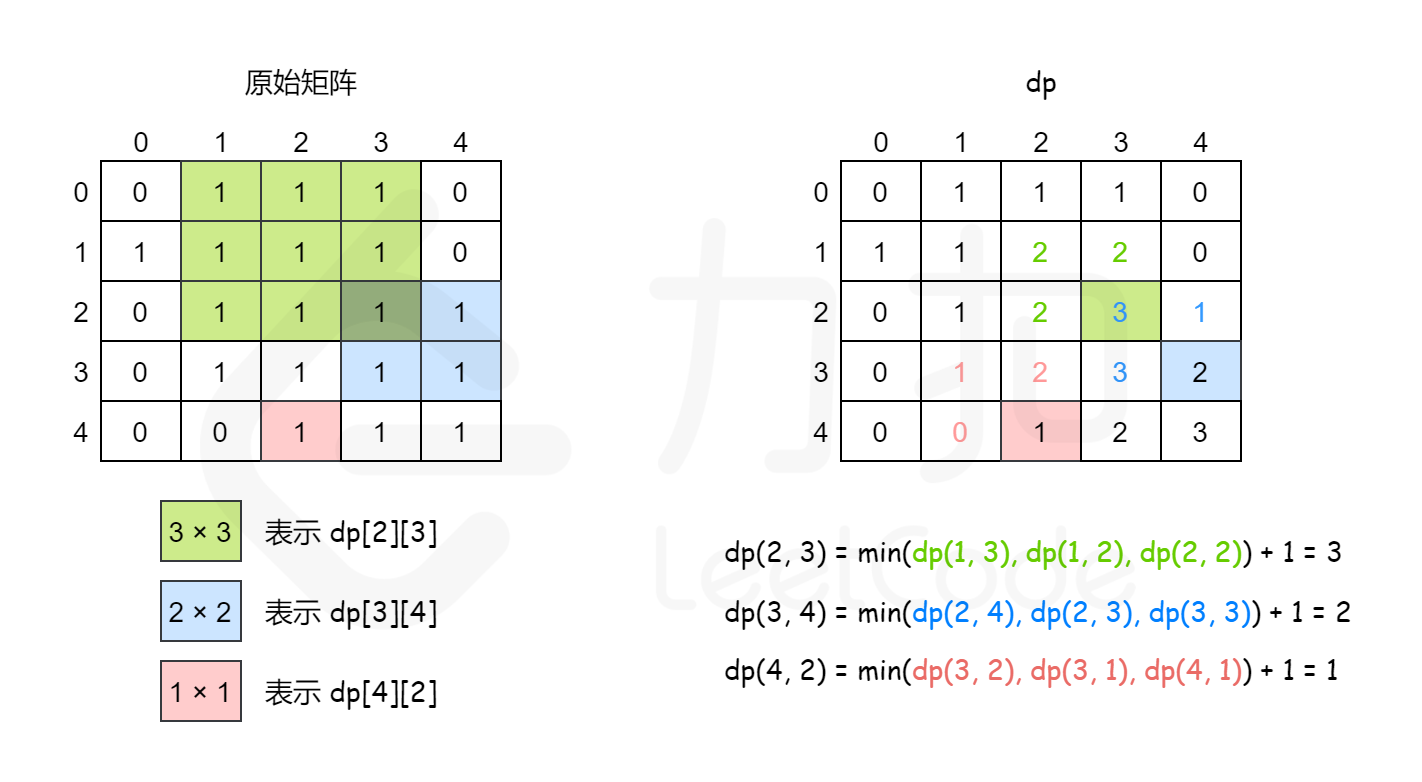•     动态规划过程：每次决策依赖于当前状态，又随即引起状态的转移。一个决策序列就是在变化的状态中产生出来的，所以，这种多阶段最优化决策解决问题的过程就称为动态规划。     假设问题是由交叠的子问题所...
一、基本概念
把多阶段过程转化为一系列单阶段问题，利用各阶段之间的关系，逐个求解。
动态规划过程：每次决策依赖于当前状态，又随即引起状态的转移。一个决策序列就是在变化的状态中产生出来的，所以，这种多阶段最优化决策解决问题的过程就称为动态规划。
假设问题是由交叠的子问题所构成，我们就能够用动态规划技术来解决它。一般来说，这种子问题出自对给定问题求解的递推关系中，这个递推关系包括了同样问题的更小子问题的解。动态规划法建议，与其对交叠子问题一次重新的求解，不如把每一个较小子问题仅仅求解一次并把结果记录在表中（动态规划也是空间换时间的）。这样就能够从表中得到原始问题的解。
二、基本思想与策略
基本思想与分治法类似，也是将待求解的问题分解为若干个子问题（阶段），按顺序求解子阶段，前一子问题的解，为后一子问题的求解提供了实用的信息。
在求解任一子问题时，列出各种可能的局部解，通过决策保留那些有可能达到最优的局部解，丢弃其它局部解。依次解决各子问题，最后一个子问题就是初始问题的解。
因为动态规划解决的问题多数有重叠子问题这个特点。为降低反复计算。对每个子问题仅仅解一次，将其不同阶段的不同状态保存在一个二维数组中。
与分治法最大的区别是：适合于用动态规划法求解的问题，经分解后得到的子问题往往不是互相独立的（即下一个子阶段的求解是建立在上一个子阶段的解的基础上，进行进一步的求解）。
三、适用的情况
能采用动态规划求解的问题的一般要具有3个性质：
（1）最优化原理：假设问题的最优解所包括的子问题的解也是最优的，就称该问题具有最优子结构，即满足最优化原理。
（2）无后效性：即某阶段状态一旦确定。就不受这个状态以后决策的影响。也就是说，某状态以后的过程不会影响曾经的状态。仅仅与当前状态有关；
（3）有重叠子问题：即子问题之间是不独立的，一个子问题在下一阶段决策中可能被多次使用到（该性质并非动态规划适用的必要条件，可是假设没有这条性质，动态规划算法同其它算法相比就不具备优势）。
四、求解的基本步骤
动态规划所处理的问题是一个多阶段决策问题，一般由初始状态开始，通过对中间阶段决策的选择，达到结束状态。这些决策形成了一个决策序列，同时确定了完成整个过程的一条活动路线(通常是求最优的活动路线)。

初始状态→│决策１│→│决策２│→…→│决策ｎ│→结束状态

(1)划分阶段：按照问题的时间或空间特征，把问题分为若干个阶段。在划分阶段时，注意划分后的阶段一定要是有序的或者是可排序的，否则问题就无法求解
(2)确定状态和状态变量：将问题发展到各个阶段时所处于的各种客观情况用不同的状态表示出来。当然，状态的选择要满足无后效性。
(3)确定决策并写出状态转移方程：状态转移就是根据上一阶段的状态和决策来导出本阶段的状态。如果确定了决策，状态转移方程也就可写出。但事实上常常是反过来做，根据相邻两个阶段的状态之间的关系来确定决策方法和状态转移方程。
(4)寻找边界条件：给出的状态转移方程是一个递推式，需要一个递推的终止条件或边界条件。
只要解决问题的阶段、状态和状态转移决策确定了，就可以写出状态转移方程（包括边界条件）。

确定状态：最后一步和转化子问题
确定转移方程
确定边界情况和初始条件
确定好计算顺序

五、常见动态规划问题
1、找零钱问题（求最值动态规划）
有面值为1元、2元和7元的硬币若干枚，如何用最少的硬币凑够27元？

1.确定状态

最后一步：（最优策略中使用的最后一枚硬币ak）
转化子问题：最少的硬币拼出更小的面值27-ak

2.转移方程
f[X] = min{f[X-2]+1 , f[X-5]+1 , f[x-7]+1}
3.初始条件和边界情况
f = 0,如果不能拼出Y,f[Y] = 正无穷
4.计算顺序
f,f,f, …
代码实现

class Solution:
def coinChange(self, coins: List[int], amount: int) -> int:
# 开数组 27 个位置的数组
f = [float("inf")] * (amount+1)
# 初始条件：0元0种方法
f = 0
n = len(coins)
#f,f....f
#每个数组空间装当前零钱最优的拼凑结果
for i in range(1,amount+1):
#对每种零钱进行遍历
#转移方程f[X] = min{f[X-2]+1 , f[X-5]+1 , f[x-7]+1}
for j in range(0,n):
if(i>=coins[j]):
f[i] = min(f[i-coins[j]]+1,f[i])
#如果凑不出目标数，返回-1
if f[-1] == float("inf"):
return -1
#返回次数
else:return f[-1]

2、走方格问题（计数型动态规划）
给定m行和n列网格，有一个机器人从左上角(0,0)出发，每一步可以向下或向右走一步，问多少种不同的方式走到右下角
1.确定状态

最后一步：
无论机器人用何种方式到达右下角，总有最后挪动的一步-向右或者向下
右下角设为（m-1,n-1）,机器人在前一步一定是（m-2,n-2）
子问题：
机器人有X种方式从左上角走到（m-2,n-1），Y种方式从左上角走到（m-1,n-2），则一共有X+Y种方式走到（m-1,n-1），一次类推
两个变量，二维数组：设f[i][j]为机器人有多少种方式从左上角走到(i , j)

2.转移方程：对任意一个格子：f[i][j] = f[i-1][j]+f[i][j-1]
3.初始条件和边界情况：

初始条件：f = 1，只有一种方法到左上角
边界情况：i = 0,j=0的时候，则前一步只有一个方向过来f[i][]j] = 1

4.计算顺序求f[m-1][n-1]（时间复杂度：O(MN)  空间复杂度(数组大小)：O(MN)）
5.代码实现
import numpy as np
def uniquePaths(m,n):   #m:行 ,n:列 5 4
#如果用(*m)*n会涉及到拷贝问题
f = np.zeros((m,n),dtype=np.int)
for j in range(n):
for i in range(m):
#边界为0的情况下均为1步
if(i==0 or j==0):
f[i][j] = 1
#转移方程
else:
f[i][j] = f[i-1][j]+f[i][j-1]
return f[i][j]
uniquePaths(5,4)

3、青蛙过桥（存在型动态规划）
有n块石头分别在x轴的0,1，2，… ，n-1位置，一只青蛙在石头0处，如果青蛙在第i块石头上，它最多往右跳ai个石头。
问青蛙是否能够调到石头n-1处
1.确定状态

最后一步：青蛙能到达石头i；石头i到石头n-1的距离(即最后一步的距离)不能超过可跳跃的最大距离即：n-1-i<=a[i]
子问题：这样原问题青蛙能否跳到石头n-1转化为规模更小(i<n-1)的子问题青蛙能不能跳到石头i。
状态：dp[j] = 青蛙能不能跳到石头j

2.转移方程：
设状态为dp[j] = 青蛙能不能跳到石头j，有：3.初始条件和边界情况
初始条件：dp = True，青蛙一开始就在石头0，无边界情况。
4.计算顺序
由初始条件dp = True，
计算dp,dp,…,dp[n-1]，结果：dp[n-1]
时间复杂度：O(n^2)（用贪心算法复杂度为O(n)），空间复杂度：O(n)
5.代码实现


展开全文• - 动态规划思想 - 子序列与子数组 - 字符串 - 若干不相邻的数和最大 - 零钱兑换 - 股票买卖最佳时机 - 等差数列 - 图dp
  动态规划其实是运筹学的一种最优化方法，动态规划问题的一般形式就是求最值。求解动态规划的核心问题是穷举。因为要求最值，肯定要把所有可行的答案穷举出来，然后在其中找最值。但是，动态规划的穷举有点特别，因为这类问题存在重叠子问题，如果暴力穷举的话效率会极其低下，所以需要「备忘录」或者「DP table」来优化穷举过程，避免不必要的计算。而且，动态规划问题一定会具备最优子结构，才能通过子问题的最值得到原问题的最值。另外，虽然动态规划的核心思想就是穷举求最值，但是问题可以千变万化，穷举所有可行解其实并不是一件容易的事，只有列出正确的「状态转移方程」才能正确地穷举。

最优性原理：

多阶段决策过程的最优决策序列具有这样的性质：不论初始状态和初始决策如何，对于前面决策所造成的某一状态而言，其后各阶段的决策序列必须构成最优策略。
例子：如果给定从A到C的最优路线，那么从最优路线上任意一点B到C的路线Ⅱ必须是由B到C的最优路线。

动态规划常常适用于有重叠子问题和最优子结构性质的问题。若要解一个给定问题，我们需要解其不同部分（即子问题），再根据子问题的解以得出原问题的解，动态规划往往用于优化递归问题。动态规划法仅仅解决每个子问题一次，具有天然剪枝的功能，从而减少计算量，一旦某个给定子问题的解已经算出，则将其记忆化存储，以便下次需要同一个子问题解之时直接查表。

动态规划步骤
1.确定动态规划状态

是否存在状态转移
什么样的状态比较好转移，找到对求解问题最方便的状态转移

2. 写出状态转移方程

使用数学归纳法思维，写出准确的状态方程
如果不能很快得出递推公式，可以先尝试一步一步把前面几步写出来，如果还是不行很可能就是 dp 数组的定义不够恰当，需要回到第一步重新定义 dp 数组的含义；或者可能是 dp 数组存储的信息还不够，不足以推出下一步的答案，需要把 dp 数组扩大成二维数组甚至三维数组

3. 写出初始化条件

dp数组整体的初始值
dp数组(二维)i=0和j=0的地方
dp存放状态的长度，是整个数组的长度还是数组长度加一，这点需要特别注意

4. 考虑输出状态

返回dp数组中最后一个值作为输出，一般对应二维dp问题。
返回dp数组中最大的那个数字，一般对应记录最大值问题。
返回保存的最大值，一般是Maxval=max(Maxval,dp[i]) 这样的形式。

5. 考虑对时间、空间复杂度的优化

子序列与子数组
53.最大子序和【简单】
给定一个整数数组 nums ，找到一个具有最大和的连续子数组（子数组最少包含一个元素），返回其最大和。LeetCode传送门
示例：

输入：[-2,1,-3,4,-1,2,1,-5,4]
输出：6
解释：连续子数组 [4,-1,2,1] 的和最大，为 6。

思路：
1. 状态：
一维数组 $dp$。$dp[i]$ 定义为以 $num[i]$ 这个数结尾的最大子序和。
2. 状态转移方程：
$dp[i]=\begin{cases}dp[i-1]+nums[i],dp[i-1]\geq0\\nums[i], \quad\qquad\qquad dp[i-1]<0\end{cases}=\max\{dp[i-1]+nums[i],nums[i]\}$
3. 考虑初始条件：

$dp=nums$；$dp$ 长度即为 $nums$ 长度。
填表（dp）方式：i 从1到 len(dp)-1。

4. 考虑输出状态：
返回 $dp$ 数组中值最大的数。
代码：
class Solution:
def maxSubArray(self, nums: List[int]) -> int:
if not nums:return 0
dp=*len(nums)
dp=nums
for i in range(1,len(nums)):
dp[i]=max(dp[i-1]+nums[i],nums[i])
return max(dp)

class Solution:
def maxSubArray(self, nums: List[int]) -> int:
dp=nums.copy()
for i in range(1,len(nums)):
dp[i]=max(dp[i-1]+nums[i],dp[i])
return max(dp)

300.最长上升子序列【中等】
给定一个无序的整数数组，找到其中最长上升子序列的长度。LeetCode传送门
示例：

输入：[10,9,2,5,3,7,101,18]
输出：4
解释：最长的上升子序列是 [2,3,7,101]，它的长度是 4。

说明：

可能会有多种最长上升子序列的组合，你只需要输出对应的长度即可。
你算法的时间复杂度应该为 $O(n^2)$ 。

思路：
1. 状态：
一维数组 $dp$。$dp[i]$ 定义为以 $num[i]$ 这个数结尾的最长递增子序列的长度。
2. 状态转移方程：
$dp[i]=max(dp[i],dp[j]+1), \forall jnums[j]$。以$nums[i]$结尾的最长递增子序列，如果存在的话，一定是在前面找到的某个（长度最大的）最长上升子序列后加上$nums[i]$，长度相应加1。
3. 考虑初始条件：

子序列最少也是自己，所以长度为1，因此把所有的 $dp$ 初始化为1；再考虑长度问题，由于 $dp[i]$ 代表的是 $nums[i]$ 的最长子序列长度，所以 $dp$ 长度即为 $nums$ 长度。
填表（dp）方式：i 从 0 到 len(dp)-1。

4. 考虑输出状态：
返回 $dp$ 数组中值最大的数。
代码：
class Solution:
def lengthOfLIS(self, nums: List[int]) -> int:
if not nums:return 0 # 判断边界条件
dp=*len(nums)	 # 初始化dp数组状态
for i in range(1,len(nums)):
for j in range(i):
if nums[i]>nums[j]:	# 状态转移
dp[i]=max(dp[i],dp[j]+1)
return max(dp) # 确定输出状态

时间复杂度：

遍历 $dp$ 列表需要$O(N)$，计算每个$dp[i]$需要$O(N)$的时间，总复杂度是$O(N^2)$。

优化：

动态规划中，通过线性遍历来计算 $dp$ 的复杂度无法降低；
优化通过线性遍历 $[0,k)$ 区间元素来得到 $dp[k]$ ：重新设计状态定义，使整个 $dp$ 为一个排序列表；这样在计算每个 $dp[k]$ 时，就可以通过二分法遍历 $[0,k)$ 区间元素，将此部分复杂度由 $O(N)$ 降至 $O(logN)$。动态规划 + 二分查找

354.俄罗斯套娃信封问题【困难】
LeetCode传送门
给定一些标记了宽度和高度的信封，宽度和高度以整数对形式 (w, h) 出现。当另一个信封的宽度和高度都比这个信封大的时候，这个信封就可以放进另一个信封里，如同俄罗斯套娃一样。
请计算最多能有多少个信封能组成一组“俄罗斯套娃”信封（即可以把一个信封放到另一个信封里面）。
说明：不允许旋转信封。
示例：

输入：envelopes = [[5,4],[6,4],[6,7],[2,3]]
输出：3
解释：最多信封的个数为 3, 组合为: [2,3] => [5,4] => [6,7]。

思路： 排序+最长上升子序列（最长递增子序列的二维问题）

Step1：按 w 进行升序排序，若 w 相同则按 h 降序排序。
Step2：对 h 进行 LIS 算法（最长上升子序列）。

代码：
class Solution:
def maxEnvelopes(self, envelopes: List[List[int]]) -> int:
envelopes.sort(key=lambda x:(x,-x))
def lis(nums):
if not nums:return 0
dp=*len(nums)
for i in range(1,len(nums)):
for j in range(i):
if nums[i]>nums[j]:
dp[i]=max(dp[i],dp[j]+1)
return max(dp)
return lis([i for i in envelopes])

from bisect import bisect_left
class Solution:
def maxEnvelopes(self, envelopes: List[List[int]]) -> int:
envelopes.sort(key=lambda x: (x, -x))
def lis(nums):
dp = []
for i in range(len(nums)):
idx = bisect_left(dp, nums[i])
if idx == len(dp):
dp.append(nums[i])
else:
dp[idx] = nums[i]
return len(dp)
return lis([i for i in envelopes])


bisect — 数组二分查找算法
这个模块对有序列表提供了支持，使得他们可以在插入新数据仍然保持有序。
bisect.bisect_left(a, x, lo=0, hi=len(a))：在 a 中找到 x 合适的插入点以维持有序。如果 x 已经在 a 里存在，那么插入点会在已存在元素之前（也就是左边）。

674.最长连续递增序列【简单】
给定一个未经排序的整数数组，找到最长且连续的的递增序列，并返回该序列的长度。LeetCode传送门
示例：

输入： [1,3,5,4,7]
输出： 3
解释: 最长连续递增序列是 [1,3,5], 长度为3。尽管 [1,3,5,7] 也是升序的子序列, 但它不是连续的，因为5和7在原数组里被4隔开。

思路：
674与300最大的不同就是连续两个字，这样就让这个问题简单很多了，因为如果要求连续的话，那么就不需要遍历两遍数组，只需要比较前后的值是不是符合递增的关系。
1. 状态：
一维数组 $dp$。$dp[i]$ 定义为以 $num[i]$ 结尾的最长连续递增子序列的长度。
2. 状态转移方程：
$dp[i]=\begin{cases}dp[i-1]+1,nums[i]>nums[i-1]\\1,\qquad\qquad\quad nums[i]\leq nums[i-1]\end{cases}$
3. 考虑初始条件：

子序列最少也是自己，所以长度为1，因此把所有的 $dp$ 初始化为1；由于 $dp[i]$ 代表的是 $nums[i]$ 的最长子序列长度，所以 $dp$ 长度即为 $nums$ 长度。
填表（dp）方式：i从1到 len(dp)-1。

4. 考虑输出状态：
返回 $dp$ 数组中值最大的数。
代码：
class Solution:
def findLengthOfLCIS(self, nums: List[int]) -> int:
if not nums:return 0
dp=*len(nums)
for i in range(1,len(nums)):
if nums[i]>nums[i-1]:
dp[i]=dp[i-1]+1
return max(dp)

字符串
5.最长回文子串【中等】
给定一个字符串 s，找到 s 中最长的回文子串。你可以假设 s 的最大长度为 1000。LeetCode传送门
示例 ：

输入：“babad”
输出：“bab”
注意: “aba” 也是一个有效答案。

思路1：
1. 状态：
二维数组 $dp$。$dp[i][j]$ 表示从第 $i$ 个位置到第 $j$ 个位置的子串是否是回文子串。
这个题目必须用二维的数组来记录状态，主要原因就是子串有回文的限制。用两个指针来记录子串的位置可以很好的实现子串的回文要求，又因为最后结果需要返回的是子串，这里不同于之前题目的用dp保存长度，我们必须找到具体哪个部分符合回文子串的要求。
2. 状态转移方程：
回文串的性质：

单个字符或空字符是回文串；
字符串首尾两个字符必须相等，否则肯定不是回文；
当字符串首尾两个字符相等时：如果子串是回文，整体就是回文，这里就有了动态规划的思想，出现了子问题；相反，如果子串不是回文，那么整体肯定不是。

对于字符串$s$，$s[i,j]$ 的子串是$s[i+1,j-1]$ ，

如果子串只有本身或者空串，即$j-1-(i+1)+1<2$ （整理得$j-i<3$），那肯定是回文子串了，所以，当$s[i]$ 和$s[j]$ 相等并且$j-i<3$ 时，我们可以直接得出$dp[i][j]$ 是True；
当$s[i]$ 和$s[j]$ 相等但$j-i\geq3$ 时，$dp[i][j]=dp[i+1][j-1]$；
当$s[i]$ 和$s[j]$ 不相等时，肯定不是回文串，$dp[i][j]=False$。

3. 考虑初始条件：

建立一个二维的初始状态是False的来保存状态的数组来表示$dp$，又因为考虑只有一个字符的时候肯定是回文串，所以$dp$表格的对角线$dp[i][i]$ 肯定是True。
填表（dp）方式：外层枚举结束位置，里层枚举结束位置

4. 考虑输出状态：
这里dp表示的是从i 到 j 是否是回文子串，这样一来就告诉我们子串的起始位置和结束位置，但是由于我们需要找到最长的子串，所以我们优化一下可以只记录起始位置和当前长度。
if dp[i][j]: # 只要dp[i][j]成立就表示回文子串，然后记录位置，返回有效答案
cur_len=j-i+1
if cur_len>max_len:
max_len=cur_len
start=i

代码：
class solution:
def longestPalindrome(self,s:str)->str:
# 边界条件
length=len(s)
if length<2:
return s
# 初始化
dp=[[False for _ in range(length)] for _ in range(length)]
for i in range(length):
dp[i][i]=True
# 状态转移
# 枚举方式一：外层枚举结束位置，里层枚举结束位置
max_len,start=1,0
for j in range(1,length):
for i in range(j):
if s[i]==s[j]:
if j-i<3:
dp[i][j]=True
else:
dp[i][j]=dp[i+1][j-1]
if dp[i][j]: # 当前最长子串长度、起始位置
cur_len=j-i+1
if cur_len>max_len:
max_len=cur_len
start=i
return s[start:start+max_len]

思路2：
状态定义、状态转移均与思路1相同，只是定义的变量不同。
class solution:
def longestPalindrome(self,s:str)->str:
n=len(s)
dp=[[False]*n for _ in range(n)]
ans=''
# 枚举方式二：外层枚举子串长度，内层枚举子串起始位置
for l in range(n):
for i in range(n):
j=i+l
if j>=n:
break
if l==0:
dp[i][j]=True
elif l==1:
dp[i][j]=s[i]==s[j]
else:
dp[i][j]=dp[i+1][j-1] and s[i]==s[j]
if dp[i][j] and l+1>len(ans):
ans=s[i:j+1]
return ans

516.最长回文子序列【中等】
给定一个字符串 s ，找到其中最长的回文子序列，并返回该序列的长度。可以假设 s 的最大长度为 1000 。LeetCode传送门
示例：

输入：“bbbab”
输出：4
一个可能的最长回文子序列为 “bbbb”。

思路：
1. 状态：
二维数组 $dp$。这里求的是最长子串的长度，所以我们可以直接定义一个二维的 $dp[i][j]$ 来表示字符串第 $i$ 个字符到第 $j$ 个字符的最长子串长度，子问题也就是每个子回文字符串的长度。
2. 状态转移方程：

当$s[i]$ 和$s[j]$ 相等时， $s[i+1...j-1]$这个字符串加上2就是最长回文子序列；
当$s[i]$ 和$s[j]$ 不相等时，就说明可能只有其中一个出现在s[i,j]的最长回文子序列中，只需要取$s[i-1,j-1]$ 加上$s[i]$ 或者$s[j]$ 的数值中较大的；3. 考虑初始条件：

很明显看出来的当只有一个字符的时候，最长回文子序列就是1，所以可以得到$dp[i][j]=1(i=j)$。当$i>j$ 时，不符合题目要求，不存在子序列，所以直接初始化为0。当$i 时，每次计算表中对应的值就会根据前一个状态的值来计算。
填表方式：每次遍历就是求出状态转移表右上角那些红色的值。按照一般的习惯都会先计算第一行的数值，但是当我们计算$dp[0,2]$ 的时候，我们会需要$dp[1,2]$ ，按照这个逻辑，我们就可以很容易发现遍历从下往上遍历会很方便计算。

4. 考虑输出状态：
返回$dp[-1]$。
代码：
class solution:
def longestPalindromeSubseq(self,s:str)->int:
n=len(s)
# 初始化动态规划状态转移矩阵
dp=[*n for _ in range(n)]
for i in range(n):
dp[i][i]=1
# 从右下角开始往上遍历
for i in range(n,-1,-1):
for j in range(i+1,n):
if s[i]==s[j]: # 当两个字符相等时，直接子字符串加2
dp[i][j]=dp[i+1][j-1]+2
else:			# 不相等时，取某边最长的字符
dp[i][j]=max(dp[i+1][j],dp[i][j-1])
return dp[-1]

72.编辑距离【困难】
LeetCode传送门
给你两个单词 word1 和 word2，请你计算出将 word1 转换成 word2 所使用的最少操作数 。
你可以对一个单词进行如下三种操作：

插入一个字符
删除一个字符
替换一个字符

示例 ：

输入：word1 = “horse”, word2 = “ros”
输出：3
解释：
horse -> rorse (将 ‘h’ 替换为 ‘r’)
rorse -> rose (删除 ‘r’)
rose -> ros (删除 ‘e’)

思路：
1. 状态：
二维数组$dp$。定义$dp[i][j]$ 为字符串word1长度为 i（前 i 个字母） 和字符串word2长度为 j （前 j 个字母）时，word1转化成word2所执行的最少操作次数的值。
2. 考虑初始条件：

第一行，是 word1 为空变成 word2 最少步数，就是插入操作。
第一列，是 word2 为空，需要的最少步数，就是删除操作。3. 状态转移方程：
从左上角开始填表：
对$dp[i][j]$，即将word1的前 i 个字母变成word2的前 j 个字母，实现方式：

当 $word1[i] == word2[j]$时，不需要对最后一位做任何操作，只需关心word1的前 i-1 个字母转换为word2的前 j-1 个字母的最小操作次数，即$dp[i][j] = dp[i-1][j-1]$；
当 $word1[i] != word2[j]$时，对应于可执行的三种操作，对word1有如下三种方式将其前 i 个字母变成word2的前 j 个字母：

替换操作：将$word1[i]$替换为$word2[j]$，然后将word1的前i-1个字母变成word2的前j-1个字母，即$dp[i][j]=dp[i-1][j-1]+1$；
删除操作：将$word1[i]$删除，然后将word1的前 i-1 个字母变成word2的前 j 个字母，即$dp[i][j]=dp[i-1][j]+1$；
插入操作：插入$word2[j]$，然后将word1的前 i 个字母变成word2的前 j-1 个字母，即$dp[i][j]=dp[i][j-1]+1$；
最后选取这三种操作方式的最小操作次数，即$dp[i][j] = min(dp[i-1][j-1], dp[i-1][j], dp[i][j-1]) + 1$

4. 考虑输出状态：
返回$dp[-1][-1]$。
代码：
class solution:
def minDistance(word1,word2):
n1,n2=len(word1),len(word2)
# 初始化dp
dp=[*(n2+1) for _ in range(n1+1)]
for j in range(1,n2+1): # 第一行
dp[j]=j
for i in range(1,n1+1): # 第一列
dp[i]=i
# 从左上角开始填表
for i in range(1,n1+1):
for j in range(1,n2+1):
if word1[i-1]=word2[j-1]:
dp[i][j]=dp[i-1][j-1]
else:
dp[i][j]=min(dp[i][j-1],dp[i-1][j],dp[i-1][j-1])+1
return dp[-1][-1]

32.最长有效括号【困难】
给定一个只包含 ‘(’ 和 ‘)’ 的字符串，找出最长的包含有效括号的子串的长度。LeetCode传送门
示例 ：

输入：")()())"
输出：4
解释：最长有效括号子串为 “()()”

思路：
1. 状态：
一维数组$dp$。$dp[i]$表示以第 i 个字符结尾的最长有效括号长度。
2. 状态转移方程：

当 $s[i]$ 为 (，$dp[i]$ 必然等于 0，因为不可能组成有效的括号；
当 $s[i]$ 为 )：

当 $s[i-1]$ 为 (，那么 $dp[i] = dp[i-2] + 2$；
当 $s[i-1]$ 为 ) ，则$s[i-2-dp[i-1]]~s[i-1]$为匹配好的有效括号，需要看$s[i-dp[i-1] - 1]$能否与$s[i]$匹配，即若 $s[i-dp[i-1] - 1]$ 为 (，那么可以匹配，则 $dp[i] = dp[i-1] + 2 + dp[i-dp[i-1]-2]$；

3. 考虑初始条件：

仅知道单个字符，有效括号长度为0。
调表顺序：从0到len(dp)-1。

4. 考虑输出状态：
返回dp的最大值。
代码：
class solution:
def longestValidParentheses(s):
if not s: return 0
n=len(s)
dp=*n
for i in range(n):
if i>0 and s[i]==')':
if s[i-1]=='(':
dp[i]=dp[i-2]+2
elif s[i-1]==')' and i-1-dp[i-1]>=0 and s[i-1-dp[i-1]]=='(':
dp[i]=dp[i-1]+2+dp[i-2-dp[i-1]]
return max(dp)

若干不相邻的数和最大
198.打家劫舍【简单】
LeetCode传送门
你是一个专业的小偷，计划偷窃沿街的房屋。每间房内都藏有一定的现金，影响你偷窃的唯一制约因素就是相邻的房屋装有相互连通的防盗系统，如果两间相邻的房屋在同一晚上被小偷闯入，系统会自动报警。
给定一个代表每个房屋存放金额的非负整数数组，计算你 不触动警报装置的情况下 ，一夜之内能够偷窃到的最高金额。
示例：

输入：[2,7,9,3,1]
输出：12
解释：偷窃 1 号房屋 (金额 = 2), 偷窃 3 号房屋 (金额 = 9)，接着偷窃 5 号房屋 (金额 = 1)。
偷窃到的最高金额 = 2 + 9 + 1 = 12 。

思路：
1. 状态：
一维数组$dp$。$dp[i]$偷窃前 i 间房子能得到的最高金额。
2. 状态转移方程：

如果抢了第 i 个房间，那么第 i-1 个房间肯定是不能抢的，这个时候需要再往前一间，用第 i-2 间的金额加上当前房间的金额，得到的状态转移方程是$dp[i]=dp[i-2]+nums[i]$ 。
如果没有抢第 i 个房间，那么肯定抢了第 i-1 间的金额，所以直接有$dp[i]=dp[i-1]$ 。

最后综合一下两种情况，就可以很快得到状态转移方程： $dp[i]=max(dp[i-2]+nums[i],dp[i-1])$。
3. 考虑初始条件：
初始化条件需要考虑第一个房子和第二个房子，之后的房子都可以按照规律直接求解，当我们只有一个房子的时候，自然只抢那间房子，当有两间房的时候，就抢金额较大的那间。综合起来就是$dp=nums$，$dp=max(nums,nums)$。
4. 考虑输出状态：
返回状态转移数组的最后一个值就是所求的最大偷窃金额。
代码：
class solution:
def rob(self,nums:List[int]) -> int:
if not nums:return 0
if len(nums)==1:return nums
dp=*len(nums)
dp=nums
dp=max(nums,nums)
for i in range(2,len(nums)):
dp[i]=max(dp[i-1],dp[i-2]+nums[i])
return dp[-1]

213.打家劫舍2【中等】
LeetCode传送门
你是一个专业的小偷，计划偷窃沿街的房屋，每间房内都藏有一定的现金。这个地方所有的房屋都围成一圈，这意味着第一个房屋和最后一个房屋是紧挨着的。同时，相邻的房屋装有相互连通的防盗系统，如果两间相邻的房屋在同一晚上被小偷闯入，系统会自动报警。
给定一个代表每个房屋存放金额的非负整数数组，计算你在不触动警报装置的情况下，能够偷窃到的最高金额。
示例：

输入: [1,2,3,1]
输出: 4
解释: 你可以先偷窃 1 号房屋（金额 = 1），然后偷窃 3 号房屋（金额 = 3）。
偷窃到的最高金额 = 1 + 3 = 4 。

思路：
1. 状态：
一维数组$dp$。$dp[i]$偷窃前 i 间房子能得到的最高金额。
2. 状态转移方程：
和上个题目类似，这个题目不一样的是现在所有房屋都围成一个圈，相比于上个问题又增加了一个限制，这样一来第一个房子和最后一个房子只能选择其中一个偷窃了。所有我们把这个问题拆分成两个问题：

偷窃了第一个房子，此时对应的是nums[1:] ，得到最大的金额value是v1 。
偷窃了最后一个房子，此时对应的是nums[:n-1] (其中n是所有房子的数量)，得到的最大金额value
是v2 。

最后的结果就是取这两种情况的最大值，即 max(v1,v2) 。
3. 考虑初始条件：
初始化一个房子和两个房子的情况就是$dp=nums$，$dp=max(nums,nums)$。
4. 考虑输出状态：
返回状态转移数组的最后一个值就是所求的最大偷窃金额。
代码：
class Solution:
def rob(self, nums: List[int]) -> int:
if not nums:return 0
elif len(nums)<=2:return max(nums)
def helper(nums):
if len(nums)<=2:return max(nums)
dp=*len(nums)
dp=nums
dp=max(nums,nums)
for i in range(2,len(nums)):
dp[i]=max(dp[i-1],dp[i-2]+nums[i])
return dp[-1]
return max(helper(nums[1:]),helper(nums[:-1]))

凑零钱
322.零钱兑换【中等】
LeetCode传送门
给定不同面额的硬币 coins 和一个总金额 amount。编写一个函数来计算可以凑成总金额所需的最少的硬币个数。如果没有任何一种硬币组合能组成总金额，返回 -1。
示例 ：

输入: coins = [1, 2, 5], amount = 11
输出: 3
解释: 11 = 5 + 5 + 1

思路：
1. 状态：
一维数组$dp$。$dp[i]$表示组成金额 i 需要的最少钞票数。
2. 状态转移方程：

假设我们知道$dp[i]$，即组成金额 i 最少的硬币数，最后一枚硬币的面值是 c，那么由于问题的最优子结构，转移方程应为：$dp[i]=dp[i-c]+1$；
但我们不知道最后一枚硬币的面值是多少，所以我们需要枚举每个硬币面额值 $c_1,...,c_n$，并选择其中的最小值。下列递推关系成立：$dp[i]=\min_{1,...,n}dp[i-c_j]+1,\quad i-c_j\geq0$3. 考虑初始条件：

$dp=0$
当不存在硬币时，$dp[i]=-1,\forall i$

4. 考虑输出状态：
返回$dp[amount]$。
代码：
class solution:
def coinChange(self,coins:List[int],amount:int) -> int:
dp=[float('inf')]*(amount+1)
dp=0
for coin in coins:
for x in range(coin,amount+1):
dp[x]=min(dp[x],dp[x-coin]+1)
return dp[amount] if dp[amount]!=float('inf') else -1

class Solution:
def coinChange(self, coins: List[int], amount: int) -> int:
dp=[float('inf')]*(amount+1)
dp=0
for x in range(1,amount+1):
for coin in coins:
if x-coin>=0:
dp[x]=min(dp[x],dp[x-coin]+1)
return dp[amount] if dp[amount] != float('inf') else -1

518.零钱兑换2【中等】
LeetCode传送门
给定不同面额的硬币和一个总金额。写出函数来计算可以凑成总金额的硬币组合数。假设每一种面额的硬币有无限个。
示例：

输入：amount = 5, coins = [1, 2, 5]
输出：4
解释：有四种方式可以凑成总金额:
5=5
5=2+2+1
5=2+1+1+1
5=1+1+1+1+1

思路：
1. 状态：
一维数组$dp$。$dp[i]$表示组成金额 i 的方案数。
2. 考虑初始条件：

当$amount=0$时，方案数为1，即不选任何硬币，$dp=1$。
当没有硬币时，$dp[i]=0,\forall i\neq 0$

3. 状态转移方程：

从基本情况没有硬币开始，一一添加硬币。
对于每个添加的硬币，从金额 0 到 amount 递归的计算组合数量，更新方案数：$dp[x]+=dp[x-coin]$。

4. 考虑输出状态：
返回$dp[amount]$。
代码：
class solution:
def change(self,amount:int,coins:List[int]) -> int:
dp=*(amount+1)
dp=1
for coin in coins:
for x in range(coin,amount+1):
dp[x]+=dp[x-coin]
return dp[amount]

股票买卖
121.买卖股票的最佳时机【简单】
LeetCode传送门
给定一个数组，它的第 i 个元素是一支给定股票第 i 天的价格。
如果你最多只允许完成一笔交易（即买入和卖出一支股票一次），设计一个算法来计算你所能获取的最大利润。
注意：你不能在买入股票前卖出股票。
示例 ：

输入：[7,1,5,3,6,4]
输出：5
解释：在第 2 天（股票价格 = 1）的时候买入，在第 5 天（股票价格 = 6）的时候卖出，最大利润 = 6-1 = 5 。
注意利润不能是 7-1 = 6, 因为卖出价格需要大于买入价格；同时，你不能在买入前卖出股票。

思路：
1. 状态：

一维数组$dp$。$dp[i]$表示前 i 天的最大利润。
minprice：前 i 天股票的最低价格。

2. 状态转移方程：

第 i 天卖出股票的最大收益，等于第 i 天的股票价格减去前 i 天的最低股票价格。
$dp[i]=\max\{dp[i-1],prices[i]-minprice\}$

3. 考虑初始条件：
dp大小为天数，全部初始化为0，minprice初始化为第一天的股票价格。
4. 考虑输出状态：
返回$dp[-1]$。
代码：
class Solution:
def maxProfit(self, prices: List[int]) -> int:
n=len(prices)
if n==0:return 0
dp=*n
minprice=prices
for i in range(1,n):
minprice=min(minprice,prices[i])
dp[i]=max(dp[i-1],prices[i]-minprice)
return dp[-1]

122.买卖股票的最佳时机2【简单】
LeetCode传送门
给定一个数组，它的第 i 个元素是一支给定股票第 i 天的价格。
设计一个算法来计算你所能获取的最大利润。你可以尽可能地完成更多的交易（多次买卖一支股票）。注意：你不能同时参与多笔交易（你必须在再次购买前出售掉之前的股票）。
示例 ：

输入：[7,1,5,3,6,4]
输出：7
解释：在第 2 天（股票价格 = 1）的时候买入，在第 3 天（股票价格 = 5）的时候卖出, 这笔交易所能获得利润 = 5-1 = 4 。
随后，在第 4 天（股票价格 = 3）的时候买入，在第 5 天（股票价格 = 6）的时候卖出, 这笔交易所能获得利润 = 6-3 = 3 。

思路：
1. 状态：
二维数组$dp$。$dp[i][j]$：$i\in\{0,1,...,len(prices)-1\},j\in\{0,1\}$。

$dp[i]$表示第 i 天持有现金时，前 i 天能获得的最大利润；
$dp[i]$表示第 i 天持有股票时，前 i 天能获得的最大利润。

2. 状态转移方程：

状态从持有现金（cash）开始，到最后一天我们关心的状态依然是持有现金（cash）；
每一天状态可以转移，也可以不动。状态转移用下图表示：因为不限制交易次数，除了最后一天，每一天的状态可能不变化，也可能转移。
$\begin{cases} dp[i]=\max\{dp[i-1],dp[i-1]+prices[i]\}\\ dp[i]=\max\{dp[i-1]-prices[i],dp[i-1]\} \end{cases}$

3. 考虑初始条件：
$dp=0,dp=-prices$
4. 考虑输出状态：
返回$dp[len(prices)-1]$，因为终止的时候一定是持有现金。
代码：
class Solution:
def maxProfit(self, prices: List[int]) -> int:
n=len(prices)
if n<2:return 0
dp=[*2 for _ in range(n)]
dp=-prices
for i in range(1,n):
dp[i]=max(dp[i-1],dp[i-1]+prices[i])
dp[i]=max(dp[i-1]-prices[i],dp[i-1])
return dp[-1]

123.买卖股票的最佳时机3【困难】
LeetCode传送门
给定一个数组，它的第 i 个元素是一支给定的股票在第 i 天的价格。
设计一个算法来计算你所能获取的最大利润。你最多可以完成 两笔 交易。
注意: 你不能同时参与多笔交易（你必须在再次购买前出售掉之前的股票）。
示例：

输入：[3,3,5,0,0,3,1,4]
输出：6
解释：在第 4 天（股票价格 = 0）的时候买入，在第 6 天（股票价格 = 3）的时候卖出，这笔交易所能获得利润 = 3-0 = 3 。
随后，在第 7 天（股票价格 = 1）的时候买入，在第 8 天 （股票价格 = 4）的时候卖出，这笔交易所能获得利润 = 4-1 = 3 。

思路：
1. 状态：
二维数组$dp$。$dp[i][j]$：$i\in\{0,1,...,len(prices)-1\},j\in\{0,1\}$。

$dp[i]$表示第 i 天还未开始交易时，前 i 天能获得的最大利润。
$dp[i]$表示第 i 天第一次买入一只股票时，前 i 天能获得的最大利润。
$dp[i]$表示第 i 天第一次卖出一只股票时，前 i 天能获得的最大利润。
$dp[i]$表示第 i 天第二次买入一只股票时，前 i 天能获得的最大利润。
$dp[i]$表示第 i 天第二次卖出一只股票时，前 i 天能获得的最大利润。

2. 状态转移方程：
“状态转移方程”可以用下面的图表示，它的特点是：状态要么停留，要么向后面走，状态不能回退。$\begin{cases} dp[i]=0\\ dp[i]=\max\{dp[i-1],dp[i-1]-prices[i]\}\\ dp[i]=\max\{dp[i-1],dp[i-1]+prices[i]\}\\ dp[i]=\max\{dp[i-1],dp[i-1]-prices[i]\}\\ dp[i]=\max\{dp[i-1],dp[i-1]+prices[i]\} \end{cases}$
3. 考虑初始条件：

第一天什么都不操作：$dp=0$，第一天第一次买入一只股票：$dp=-prices$；
第一天不可能出现状态2，3，4，赋值为一个不可能的数：$dp=dp=dp=-float('inf')$。
其他的值在状态转移时都会更新，可随意初始化，比如初始化为-float(‘inf’)。

4. 考虑输出状态：
输出状态为持有现金时的最大利润，即状态为0，2,4时的最大利润。
代码：
class Solution:
def maxProfit(self, prices: List[int]) -> int:
n=len(prices)
if n<2:return 0
dp=[[-float('inf')]*5 for _ in range(n)]
dp=0
dp=-prices
for i in range(1,n):
dp[i]=0
dp[i]=max(dp[i-1],dp[i-1]-prices[i])
dp[i]=max(dp[i-1],dp[i-1]+prices[i])
dp[i]=max(dp[i-1],dp[i-1]-prices[i])
dp[i]=max(dp[i-1],dp[i-1]+prices[i])
return max(dp[-1],max(dp[-1],dp[-1]))

188.买卖股票的最佳时机4【困难】
LeetCode传送门
给定一个数组，它的第 i 个元素是一支给定的股票在第 i 天的价格。
设计一个算法来计算你所能获取的最大利润。你最多可以完成 k 笔交易。
注意: 你不能同时参与多笔交易（你必须在再次购买前出售掉之前的股票）。
示例 ：

输入: [2,4,1], k = 2
输出: 2
解释: 在第 1 天 (股票价格 = 2) 的时候买入，在第 2 天 (股票价格 = 4) 的时候卖出，这笔交易所能获得利润 = 4-2 = 2 。

思路：
1. 状态：
三维数组$dp$。$dp[i][j][K]$ ：第 i 天之前进行 j 笔交易目前拥有 K 只股票的最大利润。

$i\in\{0,1,...,len(prices)-1\}$
$j\in\{0,1,...,k\}$
$K\in\{0,1\}$：持有股票数量只能是0或1。

2. 状态转移方程：第 i 天已经交易 j 次且目前拥有0只股票的最大利润$dp[i][j]$：前一天同状态，或者，前一天交易了 j 次且拥有1只股票，第 i 天卖出。
第 i 天已经交易 j 次且目前拥有1只股票的最大利润$dp[i][j]$：前一天同状态，或者，前一天已经交易 j-1 次且拥有0只股票，第 i 天买入一只股票。
$\begin{cases} dp[i][j]=\max\{dp[i-1][j],dp[i-1][j]+prices[i]\}\\ dp[i][j]=\max\{dp[i-1][j],dp[i-1][j-1]-prices[i]\} \end{cases}$

3. 考虑初始条件：

0次交易：

dp[i]：第i天之前进行0笔交易目前拥有0只股票的最大利润是0，即dp[i]=0；
dp[i]：第i天之前进行0笔交易目前拥有1只股票的最大利润，初始状态为现金，这种情况不会出现，可以赋值为任何值，之后的状态转移也不会用到。

第一天进行 j （j>0）笔交易：同一天买入又卖出多次

dp[j]=0
dp[j]= -prices4. 考虑输出状态：
交易次数小于等于 k 次，最优值应为所有可能交易次数下的最优值。即$dp[-1],dp[-1],...,dp[-1][k]$
代码：
class Solution:
def maxProfit(self, k, prices):
if not prices: return 0
n=len(prices)
if k >= n // 2:  # 转换成无数次交易
dp=[*2 for _ in range(n)]
dp=-prices
for i in range(1,n):
dp[i]=max(dp[i-1],dp[i-1]+prices[i])
dp[i]=max(dp[i-1]-prices[i],dp[i-1])
return dp[-1]
else:
dp = [[[None, None] for _ in range(k + 1)] for _ in range(len(prices))]
# 赋边界值
for i in range(len(prices)):
dp[i] = 0
for j in range(1, k + 1):
dp[j] = 0
dp[j] = -prices
# 状态转移
for i in range(1, len(prices)):
for j in range(1, k + 1):
dp[i][j] = max(dp[i - 1][j], dp[i - 1][j] + prices[i])
dp[i][j] = max(dp[i - 1][j], dp[i - 1][j-1] - prices[i])

# 最后从多次交易中选出最大利润
max_value= 0
for i in range(k+1):
max_value = max(max_value,dp[-1][i])
return max_value

309.最佳买卖股票时机含冷冻期【中等】
LeetCode传送门
给定一个整数数组，其中第 i 个元素代表了第 i 天的股票价格 。​
设计一个算法计算出最大利润。在满足以下约束条件下，你可以尽可能地完成更多的交易（多次买卖一支股票）:

你不能同时参与多笔交易（你必须在再次购买前出售掉之前的股票）。
卖出股票后，你无法在第二天买入股票 (即冷冻期为 1 天)。

思路：
1. 状态：
二维数组$dp$。$dp[i][j],i\in\{0,1,...,len(prices)\},j\in\{0,1,2\}$

$dp[i]$：考虑前 i 天，第 i 天持有1支股票，最大收益。
$dp[i]$：考虑前 i 天，第 i 天持有0支股票且处于冷冻期（第 i+1 天无法买入股票），最大收益。
$dp[i]$：考虑前 i 天，第 i 天持有0支股票且不处于冷冻期，最大收益。

2. 状态转移方程：

$dp[i]$：第 i-1 天就持有一支股票，或者，第 i-1 天持有0支股票且不处于冷冻期，第 i 天购买一支股票；
$dp[i]=\max\{dp[i-1],dp[i-1]-prices[i]\}$
$dp[i]$：第 i-1 天持有一支股票且在第 i-1 天卖出；
$dp[i]=dp[i]+prices[i]$
$dp[i]$：第 i-1 天不持有股票，可能处于冷冻期也可能不处于冷冻期，第 i 天不作买入或卖出操作。
$dp[i]=\max\{dp[i-1],dp[i-1]\}$

3. 考虑初始条件：
$dp=-prices,dp=0,dp=0$
4. 考虑输出状态：
$\max\{dp[-1],dp[-1]\}$
代码：
class Solution:
def maxProfit(self, prices: List[int]) -> int:
n=len(prices)
if n<2:return 0
dp=[*3 for _ in range(n)]
dp=-prices
for i in range(1,n):
dp[i]=max(dp[i-1],dp[i-1]-prices[i])
dp[i]=dp[i-1]+prices[i]
dp[i]=max(dp[i-1],dp[i-1])
return max(dp[-1],dp[-1])

714.最佳买卖股票时机含手续费【中等】
LeetCode传送门
给定一个整数数组 prices，其中第 i 个元素代表了第 i 天的股票价格 ；非负整数 fee 代表了交易股票的手续费用。
你可以无限次地完成交易，但是你每笔交易都需要付手续费。如果你已经购买了一个股票，在卖出它之前你就不能再继续购买股票了。
返回获得利润的最大值。
注意：这里的一笔交易指买入持有并卖出股票的整个过程，每笔交易你只需要为支付一次手续费。
思路：
类似122买卖股票的最佳时机2，只是在卖出股票时需要减去手续费。
代码：
class Solution:
def maxProfit(self, prices: List[int], fee: int) -> int:
n=len(prices)
if n<2:return 0
dp=[*2 for _ in range(n)]
dp=-prices
for i in range(1,n):
dp[i]=max(dp[i-1],dp[i-1]+prices[i]-fee)
dp[i]=max(dp[i-1]-prices[i],dp[i-1])
return dp[-1]

优化：
class Solution(object):
def maxProfit(self, prices, fee):
cash, hold = 0, -prices
for i in range(1, len(prices)):
cash = max(cash, hold + prices[i] - fee)
hold = max(hold, cash - prices[i])
return cash

剑指63.股票的最大利润【中等】
LeetCode传送门
假设把某股票的价格按照时间先后顺序存储在数组中，请问买卖该股票一次可能获得的最大利润是多少？
思路：
1. 状态：
一维数组$dp$。$dp[i]$表示前 i 日的最大利润 。
2. 状态转移方程：
由于题目限定 “买卖该股票一次” ，因此前 i 日最大利润 $dp[i]$ 等于前 i−1 日最大利润 $dp[i-1]$ 和第 i 日卖出的最大利润中的最大值。
$dp[i]=\max\{dp[i-1],prices[i]-\min(prices[:i])\}$
3. 考虑初始条件：
$dp=0$。
4. 考虑输出状态：
返回$dp[-1]$。
代码：
class Solution:
def maxProfit(self, prices: List[int]) -> int:
n=len(prices)
if n<2:return 0
dp=*n
minprice=prices
for i in range(1,n):
minprice=min(minprice,prices[i])
dp[i]=max(dp[i-1],prices[i]-minprice)
return dp[-1]

优化：
class Solution:
def maxProfit(self, prices: List[int]) -> int:
cost, profit = float("+inf"), 0
for price in prices:
cost = min(cost, price)
profit = max(profit, price - cost)
return profit

等差数列
413.等差数列划分【中等】
LeetCode传送门
数组 A 包含 N 个数，且索引从0开始。数组 A 的一个子数组划分为数组 (P, Q)，P 与 Q 是整数且满足 0<=P<Q<N 。
如果满足以下条件，则称子数组(P, Q)为等差数组：元素 A[P], A[p + 1], …, A[Q - 1], A[Q] 是等差的。并且 P + 1 < Q。
函数要返回数组 A 中所有为等差数组的子数组个数。
思路：
1. 状态：
一维数组$dp$。$dp[i]$表示以第 i 个元素结尾的等差数列的个数 。
2. 状态转移方程：

如果区间 (i, j) 是等差数列，那么当 A[j+1] 和 A[j] 的差值和之前的差值相等的情况下，区间 (i,j+1) 也构成一个等差数列。此外，如果区间 (i,j) 就不是一个等差数列，那么之后再向右拓展也不可能是一个等差数列了。
倘若 $A[i]-A[i-1]==A[i-1]-A[i-2]$，以 $A[i-1]$结尾的等差数列加上元素 $A[i]$，都可以重新构成等差数列，除此之外，$A[i-2],A[i-1],A[i]$也是一个等差数列。

$dp[i]=\begin{cases} dp[i-1]+1,\quad A[i]-A[i-1]==A[i-1]-A[i-2]\\ 0,\qquad \qquad \qquad else \end{cases}$
3. 考虑初始条件：
$dp=0$，带下与数组A相同。
4. 考虑输出状态：
返回$sum(dp)$。
代码：
class Solution:
def numberOfArithmeticSlices(self, A: List[int]) -> int:
dp=*len(A)
for i in range(2,len(A)):
if A[i]-A[i-1]==A[i-1]-A[i-2]:
dp[i]=dp[i-1]+1

return sum(dp)

1027. 最长等差数列【中等】
LeetCode传送门
给定一个整数数组 A，返回 A 中最长等差子序列的长度。
回想一下，A 的子序列是列表 A[i_1], A[i_2], …, A[i_k] 其中 0 <= i_1 < i_2 < … < i_k <= A.length - 1。并且如果 B[i+1] - B[i]( 0 <= i < B.length - 1) 的值都相同，那么序列 B 是等差的。
思路：
二维数组$dp$。$dp[i][d]$表示以第 i 个元素结尾、差长为 d 的等差数列的个数 。
代码：
class Solution:
def longestArithSeqLength(self, A: List[int]) -> int:
dp=[{} for _ in range(len(A))]
max_ans=1
for i in range(1,len(A)):
for j in range(i):
dp[i][A[i]-A[j]]=dp[j].get(A[i]-A[j],1)+1
max_ans=max(max_ans,dp[i][A[i]-A[j]])
return max_ans

图
62.不同路径【中等】
LeetCode传送门
一个机器人位于一个 m x n 网格的左上角 （起始点在下图中标记为“Start” ）。
机器人每次只能向下或者向右移动一步。机器人试图达到网格的右下角（在下图中标记为“Finish”）。
问总共有多少条不同的路径？思路1： 排列组合
机器到底右下角，向下几步，向右几步都是固定的，即向右走$m-1$步，向下走$n-1$步，总共需要走$m+n-2$步。我们在$m+n-2$个位置中选 $m-1$个位置向右走，即得到一个路径，故总路径数为$C_{m+n-2}^{m-1}$。
代码：
def uniquePaths(self, m: int, n: int) -> int:
return int(math.factorial(m+n-2)/math.factorial(m-1)/math.factorial(n-1))

思路2： 动态规划
1. 状态：
二维数组$dp$。$dp[i][j]$表示从左上角到$(i,j)$的路径数。
2. 状态转移方程：
$dp[i][j]=dp[i-1][j]+dp[i][j-1]$
3. 考虑初始条件：
对于第一行 $dp[j]$，或者第一列 $dp[i]$，由于都是在边界，所以只能为 1。
4. 考虑输出状态：
返回$dp[-1][-1]$。
代码：
class Solution:
def uniquePaths(self, m: int, n: int) -> int:
dp = [*n] + [+ * (n-1) for _ in range(m-1)]
for i in range(1, m):
for j in range(1, n):
dp[i][j] = dp[i-1][j] + dp[i][j-1]
return dp[-1][-1]

优化：
只需左边一列和上边一行。
class Solution:
def uniquePaths(self, m: int, n: int) -> int:
pre =  * n
cur =  * n
for i in range(1, m):
for j in range(1, n):
cur[j] = pre[j] + cur[j-1]
pre = cur[:]
return pre[-1]

63.不同路径2【中等】
LeetCode传送门
一个机器人位于一个 m x n 网格的左上角 （起始点在下图中标记为“Start” ）。
机器人每次只能向下或者向右移动一步。机器人试图达到网格的右下角（在下图中标记为“Finish”）。
现在考虑网格中有障碍物。那么从左上角到右下角将会有多少条不同的路径？
网格中的障碍物和空位置分别用 1 和 0 来表示。
说明：m 和 n 的值均不超过 100。
示例：

输入:
[
[0,0,0],
[0,1,0],
[0,0,0]
]
输出: 2
解释:
3x3 网格的正中间有一个障碍物。
从左上角到右下角一共有 2 条不同的路径：

向右 -> 向右 -> 向下 -> 向下
向下 -> 向下 -> 向右 -> 向右

思路：
1. 状态：
二维数组$dp$。$dp[i][j]$表示从左上角到$(i,j)$的路径数。
2. 状态转移方程：

如果网格$(i,j)$上有障碍物，则这个点不能到达，$dp[i][j]=0$；
如果网格$(i,j)$上没有障碍物，则可从左方或上方走过来。

$dp[i][j]=\begin{cases} dp[i-1][j]+dp[i][j-1],\quad (i,j)上无障碍物\\ 0,\qquad\qquad\qquad\qquad\qquad\quad (i,j)上有障碍物 \end{cases}$
3. 考虑初始条件：
对于第一行 $dp[j]$，或者第一列 $dp[i]$，有障碍初始化为1，无障碍初始化为0。
4. 考虑输出状态：
返回$dp[-1][-1]$。
代码：
class Solution:
def uniquePathsWithObstacles(self, obstacleGrid: List[List[int]]) -> int:
if obstacleGrid==1 or obstacleGrid[-1][-1]==1:
return 0
m,n=len(obstacleGrid),len(obstacleGrid)
dp = [[0 for _ in range(n)] for _ in range(m)]
dp = 1
for i in range(1, m):
if obstacleGrid[i] == 0:
dp[i] = dp[i-1]
for j in range(1, n):
if obstacleGrid[j] == 0:
dp[j] = dp[j-1]
for i in range(1, m):
for j in range(1, n):
if obstacleGrid[i][j] == 1:
dp[i][j] = 0
else:
dp[i][j] = dp[i - 1][j] + dp[i][j - 1]
return dp[-1][-1]

64.最小路径和【中等】
LeetCode传送门
给定一个包含非负整数的 m x n 网格，请找出一条从左上角到右下角的路径，使得路径上的数字总和为最小。
说明：每次只能向下或者向右移动一步。
示例：

输入:
[
[1,3,1],
[1,5,1],
[4,2,1]
]
输出: 7
解释: 因为路径 1→3→1→1→1 的总和最小。

思路：
1. 状态：
二维数组$dp$。$dp[i][j]$ 表示从左上角出发到 $(i,j)$ 位置的最小路径和。
2. 状态转移方程：

$i>0$且$j=0$：$dp[i]=dp[i-1]+grid[i]$；
$i=0$且$j>0$：$dp[j]=dp[j-1]+grid[j]$；
$i>0$且$j>0$：$dp[i][j]=\min\{dp[i-1][j],dp[i][j-1]\}+grid[i][j]$。

3. 考虑初始条件：
$dp=grid$。
4. 考虑输出状态：
返回$dp[-1][-1]$。
代码：
class Solution:
def minPathSum(self, grid: List[List[int]]) -> int:
for i in range(len(grid)):
for j in range(len(grid[i])):
if i==j==0:continue
elif i==0:grid[i][j]+=grid[i][j-1]
elif j==0:grid[i][j]+=grid[i-1][j]
else:grid[i][j]+=min(grid[i-1][j],grid[i][j-1])
return grid[-1][-1]

120.三角形最小路径和【中等】
LeetCode传送门
给定一个三角形，找出自顶向下的最小路径和。每一步只能移动到下一行中相邻的结点上。
相邻的结点 在这里指的是 下标 与 上一层结点下标 相同或者等于 上一层结点下标 + 1 的两个结点。
例如，给定三角形：
[
,
[3,4],
[6,5,7],
[4,1,8,3]
]
自顶向下的最小路径和为 11（即，2 + 3 + 5 + 1 = 11）。
说明：如果你可以只使用 O(n) 的额外空间（n 为三角形的总行数）来解决这个问题，那么你的算法会很加分。
思路1：
1. 状态：
二维数组$dp$。$dp[i][j]$ 表示从三角形顶部走到位置 $(i, j)$ 的最小路径和。
2. 状态转移方程：
由于每一步只能移动到下一行相邻的节点上，因此要想走到位置 $(i, j)$ ，上一步就只能在位置$(i-1, j-1)$ 或者位置 $(i-1, j)$ 。
$dp[i][j]=\min\{dp[i-1][j],dp[i-1][j-1]\}+triangle[i][j]$
边界：第 i 行有 i+1 个元素，它们对应的 j 的范围为 [0, i]。

$j=0$：$dp[i]=dp[i-1]+triangle[i]$；
$j=i$：$dp[i][i]=dp[i-1][i-1]+triangle[i][i]$

3. 考虑初始条件：
$dp=triangle$。
4. 考虑输出状态：
返回$dp[-1][-1]$。
代码：
class Solution:
def minimumTotal(self, triangle: List[List[int]]) -> int:
n = len(triangle)
f = [ * n for _ in range(n)]
f = triangle

for i in range(1, n):
f[i] = f[i - 1] + triangle[i]
for j in range(1, i):
f[i][j] = min(f[i - 1][j - 1], f[i - 1][j]) + triangle[i][j]
f[i][i] = f[i - 1][i - 1] + triangle[i][i]

return min(f[n - 1])

思路2：
从下向顶遍历可以不需要考虑边界情况。
$dp[i][j]=\min\{dp[i+1][j],dp[i+1][j+1]\}+triangle[i][j]$
代码：
class Solution:
def minimumTotal(self, triangle: List[List[int]]) -> int:
for i in range(len(triangle)-2,-1,-1):
for j in range(len(triangle[i])):
triangle[i][j]+=min(triangle[i+1][j],triangle[i+1][j+1])
return triangle

174.地下城游戏【困难】
LeetCode传送门
一些恶魔抓住了公主（P）并将她关在了地下城的右下角。地下城是由 M x N 个房间组成的二维网格。我们英勇的骑士（K）最初被安置在左上角的房间里，他必须穿过地下城并通过对抗恶魔来拯救公主。
骑士的初始健康点数为一个正整数。如果他的健康点数在某一时刻降至 0 或以下，他会立即死亡。
有些房间由恶魔守卫，因此骑士在进入这些房间时会失去健康点数（若房间里的值为负整数，则表示骑士将损失健康点数）；其他房间要么是空的（房间里的值为 0），要么包含增加骑士健康点数的魔法球（若房间里的值为正整数，则表示骑士将增加健康点数）。
为了尽快到达公主，骑士决定每次只向右或向下移动一步。
编写一个函数来计算确保骑士能够拯救到公主所需的最低初始健康点数。
说明：

骑士的健康点数没有上限。
任何房间都可能对骑士的健康点数造成威胁，也可能增加骑士的健康点数，包括骑士进入的左上角房间以及公主被监禁的右下角房间。

思路：
目标：

最大路径和：路径和代表了走过路径后剩余的健康值；
最小初始健康值。

1. 状态：
二维数组$dp$。$dp[i][j]$ 表示从坐标 $(i,j)$ 到终点所需的最小初始值。当到达坐标 $(i,j)$ 时，如果此时的路径和不小于 $dp[i][j]$，就能到达终点。
2. 状态转移方程：
对于 $dp[i][j]$，我们只要关心 $dp[i][j+1]$和 $dp[i+1][j]$ 的最小值$minn$。记当前格子的值为 $dungeon[i][j]$，那么在坐标 $(i,j)$ 的初始值只要达到 $minn-dungeon[i][j]$即可。同时，初始值还必须大于等于 1。
$dp[i][j]=\max\{\min\{dp[i+1][j],dp[i][j+1]\}-dungeon[i][j],1\}$
3. 边界条件：
当 $i=n-1$ 或者 $j=m-1$时，$dp[i][j]$ 转移需要用到的 $dp[i][j+1]$和 $dp[i+1][j]$ 中有无效值，因此代码实现中给无效值赋值为极大值。特别地，$dp[n-1][m-1]$转移需要用到的 $dp[n-1][m]$和 $dp[n][m-1]$均为无效值，因此我们给这两个值赋值为 1。
4. 考虑输出状态：
返回$dp$。
代码：
class Solution:
def calculateMinimumHP(self, dungeon: List[List[int]]) -> int:
n, m = len(dungeon), len(dungeon)
BIG = 10**9
dp = [[BIG] * (m + 1) for _ in range(n + 1)]
dp[n][m - 1] = dp[n - 1][m] = 1
for i in range(n - 1, -1, -1):
for j in range(m - 1, -1, -1):
minn = min(dp[i + 1][j], dp[i][j + 1])
dp[i][j] = max(minn - dungeon[i][j], 1)

return dp

221.最大正方形【中等】
LeetCode传送门
在一个由 0 和 1 组成的二维矩阵内，找到只包含 1 的最大正方形，并返回其面积。
示例：

输入：
1 0 1 0 0
1 0 1 1 1
1 1 1 1 1
1 0 0 1 0
输出： 4

思路：
1. 状态：
二维数组$dp$。$dp[i][j]$ 表示以 $(i,j)$ 为右下角，且只包含 1 的正方形的边长最大值。
2. 状态转移方程：若对于位置 (i, j) 有 $dp[i][j] = 4$，我们将以 (i, j) 为右下角、边长为 4 的正方形涂上色，可以发现其左侧位置 (i, j - 1)，上方位置 (i - 1, j) 和左上位置 (i - 1, j - 1) 均可以作为一个边长为 4 - 1 = 3 的正方形的右下角。也就是说，这些位置的的 dp 值至少为 3，即
$dp[i][j]-1\leq \min\{dp[i-1][j],dp[i][j-1],dp[i-1][j-1]\}+1$假设 $dp[i][j - 1]$，$dp[i - 1][j]$ 和 $dp[i - 1][j - 1]$ 中的最小值为 3，也就是说，(i, j - 1)，(i - 1, j) 和 (i - 1, j - 1) 均可以作为一个边长为 3 的正方形的右下角。我们将这些边长为 3 的正方形依次涂上色，可以发现，如果位置 (i, j) 的元素为 1，那么它可以作为一个边长为 4 的正方形的右下角，dp 值至少为 4，即：
$dp[i][j]-1\geq \min\{dp[i-1][j],dp[i][j-1],dp[i-1][j-1]\}+1$
总结来说，对于每个位置 $(i, j)$，检查在矩阵中该位置的值：

如果该位置的值是 0，则 $dp[i][j]=0$，因为当前位置不可能在由 1 组成的正方形中；
如果该位置的值是 1，则 $dp[i][j]$的值由其上方、左方和左上方的三个相邻位置的 dp 值决定。具体而言，当前位置的元素值等于三个相邻位置的元素中的最小值加 1，
$dp[i][j]=\min\{dp[i-1][j],dp[i][j-1],dp[i-1][j-1]\}+1$

3. 边界条件：
如果 i 和 j 中至少有一个为 0，则以位置 $(i, j)$ 为右下角的最大正方形的边长最大只能是 1，因此 $dp[i][j]=1$。
4. 考虑输出状态：
$dp[i][j]$最大值的平方。
代码：
class Solution:
def maximalSquare(self, matrix: List[List[str]]) -> int:
if len(matrix) == 0 or len(matrix) == 0:
return 0
maxSide = 0
rows, columns = len(matrix), len(matrix)
dp = [ * columns for _ in range(rows)]
for i in range(rows):
for j in range(columns):
if matrix[i][j] == '1':
if i == 0 or j == 0:
dp[i][j] = 1
else:
dp[i][j] = min(dp[i - 1][j], dp[i][j - 1], dp[i - 1][j - 1]) + 1
maxSide = max(maxSide, dp[i][j])

maxSquare = maxSide * maxSide
return maxSquare

1277.统计全为1的正方形子矩阵【中等】
LeetCode传送门
给你一个 m * n 的矩阵，矩阵中的元素不是 0 就是 1，请你统计并返回其中完全由 1 组成的 正方形 子矩阵的个数。
示例：

输入：matrix =
[
[0,1,1,1],
[1,1,1,1],
[0,1,1,1]
]
输出：15
解释：
边长为 1 的正方形有 10 个。
边长为 2 的正方形有 4 个。
边长为 3 的正方形有 1 个。
正方形的总数 = 10 + 4 + 1 = 15.

思路：
1. 状态：
二维数组$dp$。$dp[i][j]$ 表示以 $(i,j)$ 为右下角，且只包含 1 的正方形的边长最大值。除此定义之外，$dp[i][j] = x$ 也表示以 (i, j) 为右下角的正方形的数目为 x（即边长为 1, 2, …, x 的正方形各一个）。
2. 状态转移方程：
同221.最大正方形。3. 边界条件：
如果 i 和 j 中至少有一个为 0，则以位置 $(i, j)$ 为右下角的最大正方形的边长最大只能是 1，因此 $dp[i][j]=1$。
整合边界条件的状态转移方程可写作：
$dp[i][j]=\begin{cases} matrix[i][j],\qquad\qquad\qquad\qquad\qquad\qquad\qquad\qquad if\quad i=0\quad or\quad j=0\\ 0,\qquad\qquad\qquad\qquad\qquad\qquad\qquad\qquad\qquad\qquad\quad if\quad matrix[i][j]=0\\ \min\{dp[i-1][j],dp[i][j-1],dp[i-1][j-1]\}+1,\quad otherwise \end{cases}$
4. 考虑输出状态：
所有$dp[i][j]$的和。
代码：
class Solution:
def countSquares(self, matrix: List[List[int]]) -> int:
ans = 0
rows, columns = len(matrix), len(matrix)
dp = [ * columns for _ in range(rows)]
for i in range(rows):
for j in range(columns):
if matrix[i][j] == 1:
if i == 0 or j == 0:
dp[i][j] = 1
else:
dp[i][j] = min(dp[i - 1][j], dp[i][j - 1], dp[i - 1][j - 1]) + 1
ans+=dp[i][j]

return ans

class Solution:
def countSquares(self, matrix: List[List[int]]) -> int:
m, n = len(matrix), len(matrix)
dp = [ * n for _ in range(m)]
ans = 0
for i in range(m):
for j in range(n):
if i == 0 or j == 0:
dp[i][j] = matrix[i][j]
elif matrix[i][j] == 0:
dp[i][j] = 0
else:
dp[i][j] = min(dp[i][j - 1], dp[i - 1][j], dp[i - 1][j - 1]) + 1
ans += dp[i][j]
return ans


参考
Datawhale社区开源教程之leetcode编程实践
LeetCode题解
更多阅读
动态规划系列
掌握动态规划，助你成为优秀的算法工程师
Dynamic Programming Practice Problems
浅谈什么是动态规划以及相关的「股票」算法题
有了四步解题法模板，再也不害怕动态规划!
（进阶版）有了四步解题法模板，再也不害怕动态规划！


展开全文# 动态规划经典题目pythonpython 订阅